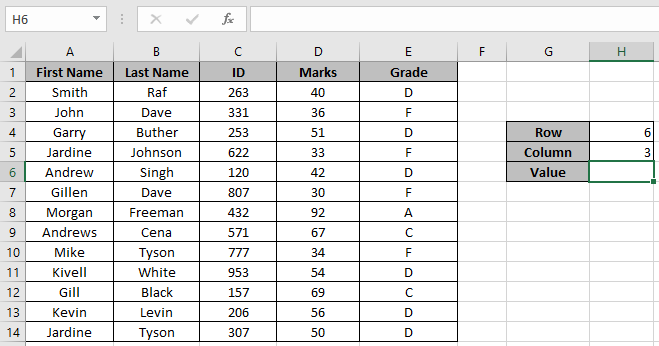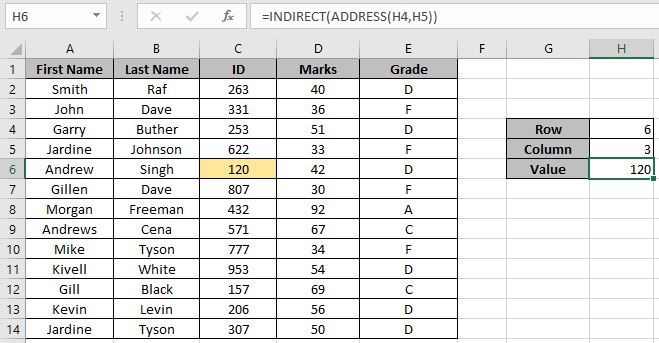Get the cell value at a given row and column number

In this article, we will learn about how to get the cell value at a given row and column number in Excel.

To get the cell value you need to know the address of the cell. But here we have the Row & column number of the cell where our required value is. So we use a combination of INDIRECT function & ADDRESS function to get the cell value.

The INDIRECT function is a cell reference function. It takes the value in the cell as address and returns the value in the address cell.

Syntax:

=INDIRECT (ref_text, [a1])

The ADDRESS function simply returns the address of a cell by using row number and column number.

Syntax:

= ADDRESS ( row_num , col_num )

Let's make a formula using the above functions:
Firstly, we can get the address of the function as ADDRESS function returns the address of the cell using row & column number. And then Indirect function extracts out the cell value from the address returned by the address function.
Syntax:

=INDIRECT ( ADDRESS ( row , col ) )

Let’s understand this function using it in an example.

Here we have table_array and Row & Column number in the right of the below snapshot.We need to find the cell value in 6th row and 3rd column

Use the formula:

INDIRECT ( ADDRESS ( H4 , H5 ) )

Explanation:
ADDRESS ( H4 , H5 ) returns \$C\$6 (address to the required cell).
The INDIRECT (\$C\$6) returns the cell value at C6 cell.As you can see the formula returns the value for a given row & column number.

Notes:

1. The function returns an error if the address returned by the ADDRESS function is not valid.
2. The function returns 0 if the cell value is blank.
3. Use the INDEX function as INDIRECT function is a volatile function.

That's all about, how to get the cell value at a given row and column number in Excel. Explore more articles on Excel Formulas here. Please feel free to state your query or feedback for the above article.

Related Articles
How to use the INDIRECT function in Excel

How to use the ADDRESS function in Excel

How to use the VLOOKUP function in Excel

How to use the HLOOKUP function in Excel